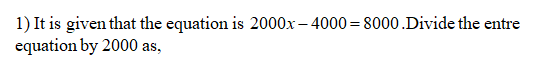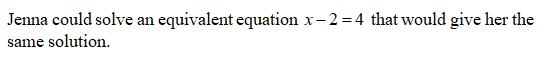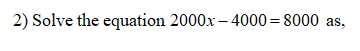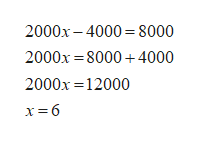# Jenna wants to solve the equation 2000x−4000=8000.What easier equation could she solve instead that would give her the same solution? (In other words, what equivalent equation has easier numbers to work with?). Justify that your equation in part (1) is equivalent to 2000x−4000=8000 by showing that they have the same solution.  Now Jenna wants to solve 350−x50=750. Write and solve an equivalent equation with easier numbers that would give her the same answer.

Question
1 views

Jenna wants to solve the equation 2000x−4000=8000.

1. What easier equation could she solve instead that would give her the same solution? (In other words, what equivalent equation has easier numbers to work with?)

2. . Justify that your equation in part (1) is equivalent to 2000x−4000=8000 by showing that they have the same solution.

3. Now Jenna wants to solve 350−x50=750. Write and solve an equivalent equation with easier numbers that would give her the same answer.

check_circle

Step 1Step 2Step 3...help_outlineImage Transcriptionclose2000x – 4000 = 8000 2000x = 8000 + 4000 2000x =12000 x= 6 fullscreen

### Want to see the full answer?

See Solution

#### Want to see this answer and more?

Solutions are written by subject experts who are available 24/7. Questions are typically answered within 1 hour.*

See Solution
*Response times may vary by subject and question.
Tagged in

### Other